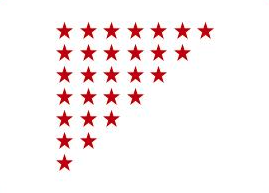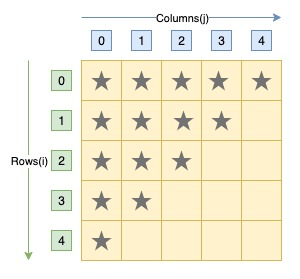# C Program to Print Inverted Right Triangle Star Pattern

Here is a C program to print a inverted right angles triangle pattern of star character using loops. For a inverted right triangle star pattern of 7 rows. Program's output should be:#### Required Knowledge

Algorithm to print inverted right triangle star pattern using loop
If you look closely, this pattern is the vertically inverted pattern of right triangle star pattern. As the row number increases from top to bottom, number of stars in a row decreases.
NOTE: The index of rows and columns starts from 0.
• Take the number of rows(N) of inverted right triangle as input from user using scanf function.
• Number of stars in Kth row is equal to (N - K + 1). In the pattern given above, N = 7. Hence, 1st row contains 7 star, 2nd row contains 6 stars, 3rd row contains 5 stars.
• We will use two for loops to print inverted right triangle star pattern.
• For a inverted right triangle star pattern of N rows, outer for loop will iterate N time(from i = 0 to N-1). Each iteration of outer loop will print one row of the pattern.
• For jth row of inverted right triangle pattern, inner loop will iterate N-j times(from j = 0 to N-j). Each iteration of inner loop will print one star character.

Here is the matrix representation of the inverted right triangle star pattern. The row numbers are represented by i whereas column numbers are represented by j.## C program to print inverted right triangle star pattern

```#include<stdio.h>

int main() {
int i,j,rows;
printf("Enter the number of rows\n");
scanf("%d", &rows);

for(i = 0; i < rows; i++) {
/* Prints one row of triangle */
for(j = 0; j < rows - i; j++) {
printf("* ");
}
/* move to next row */
printf("\n");
}
return 0;
}
```
Output
```Enter the number of rows
7
* * * * * * *
* * * * * *
* * * * *
* * * *
* * *
* *
*
```

Related Topics
 C program mirrored right triangle star pattern C program reversed right triangle star pattern C program reversed pyramid star pattern C program hollow diamond star pattern C program hut star pattern C program heart shape star pattern C program natural number triangle pattern C program same row element triangle pattern C program binary triangle pattern List of all C pattern programs